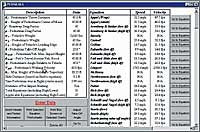Lawyers & Judges Publishing Company, Inc.# Pedestrian Formulas for Excel ®

\$ 55.00

Save many hours calculating vehicle speeds for pedestrian accidents using these pre-formatted spreadsheets. Pedestrian accident speeds can be analyzed with a comparatively large number of formulas.

However, one disadvantage arises from the increasing numbers of pedestrian formulas because no one can definitively state that any given equation is the best one to use in a particular situation.

For that reason, this program will do just that. This program simultaneously calculates and displays the values of the equations, in both mph and fps. If an equation is not applicable due to the circumstances surrounding the accident or insufficient data, that equation will display a result of "N/A", and the reason why the equation was not completed will be displayed. Pedestrian Formulas for Excel®